NEET  >  AIIMS Physics Mock Test - 7

# AIIMS Physics Mock Test - 7

Test Description

## 60 Questions MCQ Test AIIMS Mock Tests & Previous Year Papers | AIIMS Physics Mock Test - 7

AIIMS Physics Mock Test - 7 for NEET 2022 is part of AIIMS Mock Tests & Previous Year Papers preparation. The AIIMS Physics Mock Test - 7 questions and answers have been prepared according to the NEET exam syllabus.The AIIMS Physics Mock Test - 7 MCQs are made for NEET 2022 Exam. Find important definitions, questions, notes, meanings, examples, exercises, MCQs and online tests for AIIMS Physics Mock Test - 7 below.
Solutions of AIIMS Physics Mock Test - 7 questions in English are available as part of our AIIMS Mock Tests & Previous Year Papers for NEET & AIIMS Physics Mock Test - 7 solutions in Hindi for AIIMS Mock Tests & Previous Year Papers course. Download more important topics, notes, lectures and mock test series for NEET Exam by signing up for free. Attempt AIIMS Physics Mock Test - 7 | 60 questions in 60 minutes | Mock test for NEET preparation | Free important questions MCQ to study AIIMS Mock Tests & Previous Year Papers for NEET Exam | Download free PDF with solutions
 1 Crore+ students have signed up on EduRev. Have you?
AIIMS Physics Mock Test - 7 - Question 1

### An ac source is rated at 220V, 50Hz. The time taken for voltage to change from its peak value to zero is

AIIMS Physics Mock Test - 7 - Question 2

### X - rays which can penetrate through longer distances in substances are called

AIIMS Physics Mock Test - 7 - Question 3

### An air capacitor of capacity C = 10μ F is connected to a constant voltage battery of 12 volt. Now the space between the plates is filled with a liquid of dielectric constant 5. The charge that flows now from battery to the capacitor is

Detailed Solution for AIIMS Physics Mock Test - 7 - Question 3

C = 10 μ F
Charge in capacitor q = CV
= 10 x 12
= 120 μ C
On keeping liquid of dielectric constant5, capacity will becomes of 5 time.
∴ Charge q = 5 x 120
= 600 μ C

AIIMS Physics Mock Test - 7 - Question 4

An oxide coated filament is useful in vacuum tubes because essentially

AIIMS Physics Mock Test - 7 - Question 5

When wavelength of incident photon is decreased upon

AIIMS Physics Mock Test - 7 - Question 6

Which of the following law is used in the Millikan's method for the determination of charge?

AIIMS Physics Mock Test - 7 - Question 7

Quantum theory of light gives concept of

AIIMS Physics Mock Test - 7 - Question 8

An electrical cable having a resistance of 0.2 Ω delivers 10 kW at 200 V.D.C. to a factory. What is the efficiency of transmission?

AIIMS Physics Mock Test - 7 - Question 9

A bomb of 12 kg explodes into two pieces of masses 4 kg and 8 kg. The velocity of 8 kg mass is 6 m/sec. The kinetic energy of the other mass is

AIIMS Physics Mock Test - 7 - Question 10

A generator at a utility company produces 100 A of current at 4000 V. The voltage is stepped up to 240000 V by a transformer before it is sent on a high voltage transmission line. The current in transmission line is

AIIMS Physics Mock Test - 7 - Question 11

A rectangular, a square, a circular and an elliptical loop, all in the (x - y) plane, are moving out of a uniform magnetic field with a constant velocity,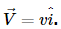The magnetic field is directed along the negative z axis direction. The induced emf, during the passage of these loops, out of the field region, will not remain constant for:

AIIMS Physics Mock Test - 7 - Question 12

Which quantity is increased in step-down transformer ?

AIIMS Physics Mock Test - 7 - Question 13

The pressure exerted by an electromegnetic wave of intensity I on a non-reflecting surface is (where c = Velocity of light)

AIIMS Physics Mock Test - 7 - Question 14

What is the value of inductance L for which the current is a maximum in series LCR circuit with C = 10 μF and ω = 1000s−1 ?

AIIMS Physics Mock Test - 7 - Question 15

An electric dipole is kept in a uniform electric field. It experiences

AIIMS Physics Mock Test - 7 - Question 16

Two capacitors of capacity 4μF and 6μF are connected in series and a battery is connected to the combination the energy stored is E1. If they are connected in parallel and if the same battery is connected to this combination the energy stored is E2. The ratio of E1 and E2 is

Detailed Solution for AIIMS Physics Mock Test - 7 - Question 16

As the capacitors are connected in series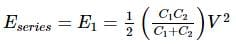As the capacitors are connected in parallel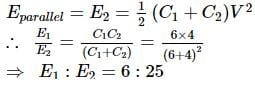AIIMS Physics Mock Test - 7 - Question 17

The same retarding force is applied to stop a train. The train stops after 80 m. If the speed is doubled, then the distance will be

AIIMS Physics Mock Test - 7 - Question 18

A light and a heavy body have equal kinetic energy. Which one has a greater momentum ?

AIIMS Physics Mock Test - 7 - Question 19

The energy emitted per second by a black body at 27ºC is 10 J. If the temperature of the black body is increased to 327ºC, the energy emitted per second will be

AIIMS Physics Mock Test - 7 - Question 20

A 4μ F conductor is charged to 400 volts and then its plates are joined through a resistance of 1 k Ω. The heat produced in the resistance is

Detailed Solution for AIIMS Physics Mock Test - 7 - Question 20

The energy stored in the capacitor = 1/2cv2

i.e. -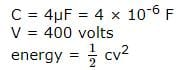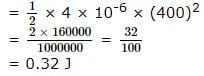This energy will be converted into heat in the resistor

AIIMS Physics Mock Test - 7 - Question 21

A certain amount of current when flowing in a properly set tangent galvanometer, produces a deflection of 45º. If the current be reduced by a factor of √3, the deflection would

AIIMS Physics Mock Test - 7 - Question 22

A steel wire of length 'L' has a magnetic moment 'M'. The wire is bent into a semi circular arc its magnetic moment will be

AIIMS Physics Mock Test - 7 - Question 23

If rope of lift breaks suddenly, the tension exerted by the surface of lift (a=acceleration of lift)

AIIMS Physics Mock Test - 7 - Question 24

The work done in stretching a wire by 1 mm is 4 J. The work done in stretching another wire of the same material, but with double the radius and half the length by 2 mm is

Detailed Solution for AIIMS Physics Mock Test - 7 - Question 24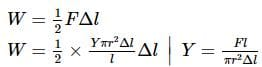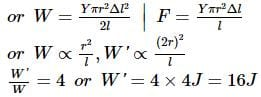AIIMS Physics Mock Test - 7 - Question 25

Beta particles are

AIIMS Physics Mock Test - 7 - Question 26

The P.E. of a simple harmonic oscillator, when the particle is half way to its end point is

AIIMS Physics Mock Test - 7 - Question 27

In the following question, a Statement of Assertion (A) is given followed by a corresponding Reason (R) just below it. Read the Statements carefully and mark the correct answer-
Assertion(A): A parallel plate capacitor is connected across battery through a key. A dielectric slab of constant K is introduced between the plates. The energy stored becomes K times.
Reason(R): The surface density of charge on the plates remains constant or unchanged.

AIIMS Physics Mock Test - 7 - Question 28

In the following question, a Statement-1 is given followed by a corresponding Statement-2 just below it. Read the statements carefully and mark the correct answer-
Statement-1:
Two cylinders, one hollow (metal) and the other solid (wood) with the same mass and identical dimensions are simultaneously allowed to roll without slipping down an inclined plane from the same height. The hollow cylinder will reach the bottom of the inclined plane first.
Statement-2:
By the principle of conservation of energy, the total kinetic energies of both the cylinders are identical when they reach the bottom of the incline.

AIIMS Physics Mock Test - 7 - Question 29

In the following question, a Statement of Assertion (A) is given followed by a corresponding Reason (R) just below it. Read the Statements carefully and mark the correct answer-
Assertion(A): Infrared radiations are used to preserve food stuffs.
Reason(R): Infrared radiations are produced by ionised gases.

AIIMS Physics Mock Test - 7 - Question 30

In the following question, a Statement of Assertion (A) is given followed by a corresponding Reason (R) just below it. Read the Statements carefully and mark the correct answer-
Assertion(A):Nuclei having number about 60 are most stable.
Reason(R):When two or more light nuclei are combined into a heavier nucleus, then the binding energy per nucleon will increase.

AIIMS Physics Mock Test - 7 - Question 31

In the following question, a Statement of Assertion (A) is given followed by a corresponding Reason (R) just below it. Read the Statements carefully and mark the correct answer-
Assertion(A): Radio waves are not electomagnetic waves.
Reason(R): Basic source of electromagnetic wave is an accelerated charge .

AIIMS Physics Mock Test - 7 - Question 32

In the following question, a Statement of Assertion (A) is given followed by a corresponding Reason (R) just below it. Read the Statements carefully and mark the correct answer-
Assertion(A):Mass of nuclear is less than the sum of the masses nucleons present in it.
Reason(R):When the nuclers to form a nucleus, some energy is liberated.

AIIMS Physics Mock Test - 7 - Question 33

In the following question, a Statement of Assertion (A) is given followed by a corresponding Reason (R) just below it. Read the Statements carefully and mark the correct answer-
Assertion(A): The linear distance travelled by the particle in the direction of magnetic field in one complete circle is called the pitch of the parth .
Reason(R): Pitch = ν sin θ x Periodic time

AIIMS Physics Mock Test - 7 - Question 34

In the following question, a Statement of Assertion (A) is given followed by a corresponding Reason (R) just below it. Read the Statements carefully and mark the correct answer-
Assertion(A): A beam of electrons passes undeflected through a region of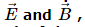Reason(R): In the region,both are present and perpendicular to each other and the particle is moving perpendicular to both of them.

AIIMS Physics Mock Test - 7 - Question 35

In the following question, a Statement of Assertion (A) is given followed by a corresponding Reason (R) just below it. Read the Statements carefully and mark the correct answer-
Assertion(A): In cold cathode emission , work function of metal is provided by a strong electric field.

AIIMS Physics Mock Test - 7 - Question 36

In the following question, a Statement of Assertion (A) is given followed by a corresponding Reason (R) just below it. Read the Statements carefully and mark the correct answer-
Assertion(A): When a Wheatstone bridge is balanced then on interchanging the positions of the galvanometer and the cell there is no deflection in the galvanometer .
Reason(R): In balance condition the ratio of the resistances of any two adjacent arms across of the bridge is equal to the ratio of the resistance of the remaining two adjacent arms.

AIIMS Physics Mock Test - 7 - Question 37

In the following question, a Statement-1 is given followed by a corresponding Statement-2 just below it. Read the statements carefully and mark the correct answer-
Statement-1:
It is easier to pull a heavy object than to push it on a level ground.
Statement-2:
The magnitude of frictional force depends on the nature of the two surfaces in contact.

AIIMS Physics Mock Test - 7 - Question 38

In the following question, a Statement of Assertion (A) is given followed by a corresponding Reason (R) just below it. Read the Statements carefully and mark the correct answer-
Assertion(A): Faraday's laws are consequences of conservation of energy.
Reason(R): In a purely resistive AC circuit, the current lags behind the emf in phase.

AIIMS Physics Mock Test - 7 - Question 39

In the following question, a Statement of Assertion (A) is given followed by a corresponding Reason (R) just below it. Read the Statements carefully and mark the correct answer-
Assertion(A): Holes in the wall of the sound board of a sonometer help in increasing the intensity of sound produced.
Reason(R): Air inside the sound board remains in communication with the air outside throught the holses.

AIIMS Physics Mock Test - 7 - Question 40

In the following question, a Statement of Assertion (A) is given followed by a corresponding Reason (R) just below it. Read the Statements carefully and mark the correct answer-
Assertion(A): γ-rays provide information about structure of atomic nuclei.
Reason (R): γ-rays are not deflected in electric or magnetic fields.

AIIMS Physics Mock Test - 7 - Question 41

In the following question, a Statement of Assertion (A) is given followed by a corresponding Reason (R) just below it. Read the Statements carefully and mark the correct answer-
Assertion(A): In an elastic collision of two billiard balls, the total kinetic energy is conserved during the short time of oscillation of the balls, when they are in contact.
Reason(R): Energy spent against friction does not follow the law of conservation of energy.

AIIMS Physics Mock Test - 7 - Question 42

In the following question, a Statement of Assertion (A) is given followed by a corresponding Reason (R) just below it. Read the Statements carefully and mark the correct answer-
Assertion(A):The parabolic curves obtained in Thomson's mass spectrograph show that ions of higher value of specific charge lie on the outer parabola.
Reason(R): Ions of different veocities arrive at different points on the parabola.

AIIMS Physics Mock Test - 7 - Question 43

In the following question, a Statement of Assertion (A) is given followed by a corresponding Reason (R) just below it. Read the Statements carefully and mark the correct answer-
Assertion(A):A soda water bottle is falling freely the bubbles of the gas will not rise in water.
Reason(R):Pressure in the water does not increase with depth.

AIIMS Physics Mock Test - 7 - Question 44

In the following question, a Statement of Assertion (A) is given followed by a corresponding Reason (R) just below it. Read the Statements carefully and mark the correct answer-
Assertion(A): The surface charge densities of two spherical conductors of radii r1 and r2 are equal. Then electric field intensities near their surfaces are also equal.
Reason(R): Surface charge density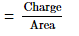AIIMS Physics Mock Test - 7 - Question 45

In the following question, a Statement-1 is given followed by a corresponding Statement-2 just below it. Read the statements carefully and mark the correct answer-
Statement-1:
For an observer looking out through the window of a fast moving train, the nearby objects appear to move in the opposite direction to the train, while the distant objects appear to be stationary.
Statement-2:
If the observer and the object are moving at velocities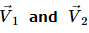respectively with reference to a laboratory frame, the velocity of the object with respect to the observer is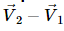AIIMS Physics Mock Test - 7 - Question 46

In the following question, a Statement of Assertion (A) is given followed by a corresponding Reason (R) just below it. Read the Statements carefully and mark the correct answer-
Assertion(A): The specific heat capacity at constant volume and at constant pressure are having different values because the system does different works against the surroundings in constant volume and constant pressure processes.
Reason(R) : From Ist law of thermodynamics, Q = dU + W where symbols have their usual meanings.

AIIMS Physics Mock Test - 7 - Question 47

An electric motor exerts a force of 40 N on a cable and pulls it by a distance of 30 m in one minute. The power supplied by the motor is

AIIMS Physics Mock Test - 7 - Question 48

A body is projected at such an angle that the horizontal range is three times the greatest height. The angle of projection is

AIIMS Physics Mock Test - 7 - Question 49

In a laboratory four convex lenses L1 , L2 , L3 and L4 of focal lengths 2, 4, 6 and 8cm, respectively are available. Two of these lenses form a telescope of length 10cm and magnifying power 4. The objective and eye lenses are respectively

AIIMS Physics Mock Test - 7 - Question 50

A person uses spectacle of power + 2 D. He is suffering from

AIIMS Physics Mock Test - 7 - Question 51

The splitting of white into several colours on passing through a glass prism is due to

AIIMS Physics Mock Test - 7 - Question 52

At 0 K temperature, a p-type semiconductor

AIIMS Physics Mock Test - 7 - Question 53

In p-type semiconductor the majority and minority charge carriers are respectively

AIIMS Physics Mock Test - 7 - Question 54

The approximate value of heat produced in 5 min. by a bulb of 210 watt is (J=4.2 joule/calorie)

AIIMS Physics Mock Test - 7 - Question 55

The unit of force is a

AIIMS Physics Mock Test - 7 - Question 56

Length cannot be measured by

AIIMS Physics Mock Test - 7 - Question 57

A wavefront is an imaginary surface where

AIIMS Physics Mock Test - 7 - Question 58

Two sources of same intensity interfere at a point and produced resultant I. When one source is removed, the intensity at that point will be

AIIMS Physics Mock Test - 7 - Question 59

The wave theory of light was given by

AIIMS Physics Mock Test - 7 - Question 60

Yoe lift a heavy book from the floor of the room and keep it in the book-shelf having a height 2m. In this process you take 5 seconds. The work done by you will depend upon

## AIIMS Mock Tests & Previous Year Papers

3 videos|41 docs|66 tests
 Use Code STAYHOME200 and get INR 200 additional OFF Use Coupon Code
Information about AIIMS Physics Mock Test - 7 Page
In this test you can find the Exam questions for AIIMS Physics Mock Test - 7 solved & explained in the simplest way possible. Besides giving Questions and answers for AIIMS Physics Mock Test - 7, EduRev gives you an ample number of Online tests for practice

## AIIMS Mock Tests & Previous Year Papers

3 videos|41 docs|66 tests

### How to Prepare for NEET

Read our guide to prepare for NEET which is created by Toppers & the best Teachers